# Understanding Electrons

## The Nature Of Electrons

Electrons are believed by many to be point particles with no substructure. They are also believed to be much smaller than a proton, but in the hydrogen atom, for example, the electron is the size of the atom. In the solid state, electrons can actually be many times larger than an atom (see Misconception Series: The Size of the Electron).

The work [1,2] of Dr. John G. Williamson, Dr. Martin B. van der Mark, and Dr. Vivian Robinson has elucidated aspects of the substructure of the electron.

An electron-positron pair is formed when two photons of the appropriate energy are condensed, forming two particles. One of the resulting double-loops with its electric field (the green spines) pointing outwards will have a positive charge — the positron, and the other, with its electric field pointing inwards, will have a negative charge — the electron. Similarly, when an electron and a positron interact, they unlock each other’s angular momenta, releasing the once-confined photons as radiation in a matter-antimatter annihilation.

Each particle contains a confined photon making two complete revolutions for every one wavelength, resulting in a phase-locked, stationary wave of toroidal topology that defines the particle.A photon (left) and the Williamson-van der Mark electron snapshot (right)

The snapshot of the electron (above, right) can be misleading because it is really many images combined. In normal space, each lens-shaped disk represents a slice through the same spherical distribution. The toroidal (donut) shape represents the phase-locked path of the rotating photon in momentum space (spatial frequency space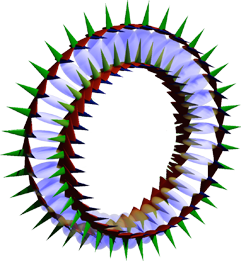). In order to conserve the circularly-polarized photon’s angular momentum, the toroid will also tumble in space like a head-over-heels spinning ring (). The sphere in the center represents the result of this — a projection onto normal 3-dimensional space of the electron’s charge distribution, which is perfectly spherical in normal “space-space” ().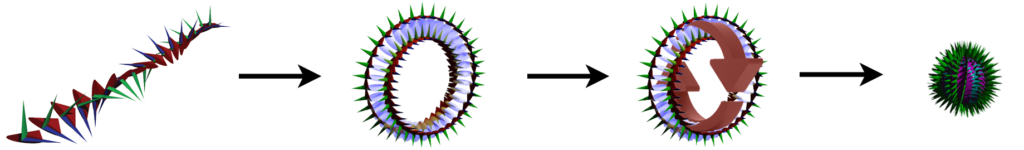The angular momentum consequences of a circularly-polarized photon forming a light-speed, double-loop rotation that is an electron.

An isolated electron is thus a self-confined knot of concentrated energy traveling around itself at the speed of light. (CLICK HERE to see one of John Williamson’s lectures on the subject.)

This revolutionary work explains (and unifies) the electron’s shared particle and wave nature, and it similarly — and notably — bridges the gap between the quantum and classical realms. While an electron is a particle, its underlying nature is electromagnetic, containing a photon, which itself has both wave and particle properties. It is held together by a powerful coherence, far stronger than the strong nuclear force, here dubbed the “super strong force.”

Electrons are harmonic stationary waves that have electric charge, magnetic field, and angular momentum, and they are affected by the charge, field, and spin of other particles in their vicinity. As a result, when multiple electrons interact in the same atom or molecule, they produce orbital regions that are symmetrical, phase-locked, resonant, coherent, spherically-harmonic, stationary electron waves. These stationary waves represent a maximum symmetry state of the system, incorporating regions of positive and negative wave interference. Note that within this new theory of quantum electromagnetism, these coherent states need not be simply the lowest energy states. Quasi-stable coherent states of higher energy may include, for example, a spin coherence (see below).

The entire atomic system forms a single, coherent, harmonic quantum state. Each electron within this state can no longer be seen as an independent particle but as an essential component of the harmonic electron stationary wave. Any addition or subtraction of energy or electrons will cause the entire system to snap into its next most stable harmonic state.

The image above is made with a rotating string, and it evokes the idea of a resonant wave in the linear direction.

This image (below) represents the 5 full electron shells of the xenon (Xe) atom, and it seeks to evoke the idea of the spherical resonance wave, a series of concentric harmonics constituting the single multi-electron state of the atom. It depicts an innermost 1st-shell sphere (not shown) within a 2nd tetrahedral shell (dark blue) within a 3rd cubic antiprismatic shell (light blue) within an anti-aligned 4th cubic antiprismatic shell (brown) within a 5th tetrahedral shell (pink). Nodal boundaries of low (though non-zero) electron density in electric field space separate each shell, as well as each orbital “slice” within each shell.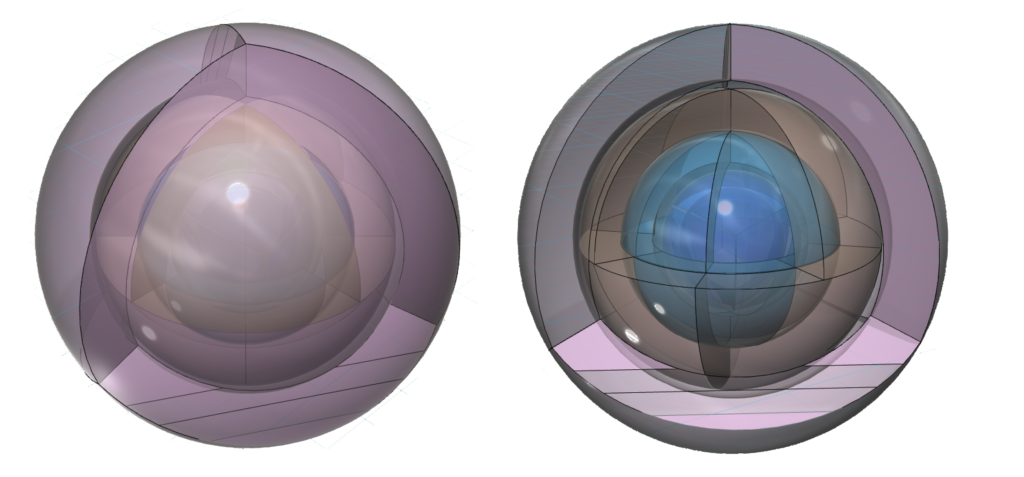Xenon’s 5 full shells (left), and with orbitals removed (right) for ease of viewing.

Within each orbital slice, electron density will be highest at the center of the face of each orbital and will decrease toward the nodal regions that divide the orbitals, where electron density will be lowest (though not necessarily zero).

## Electrons In Atoms

NUCLEAR ATTRACTION
Electrons are present because they are attracted into the positive charge well of the nuclear protons. As electrons arrive, they will try to approach and envelop the nucleus with a simple spherical symmetry. In the hydrogen (H) atom, its single electron creates a spherical s-orbital. The helium (He) nucleus attracts two electrons, which merge into the di-electron state (see below) and are thus both able to surround the nucleus in the same (superimposed) s-orbital symmetry. If there is already a spherical di-electron shell, new electrons will be impeded from approaching the nucleus. They will therefore cluster around the core electrons in a symmetrical fashion, forming a second shell around the first — a single-electron second shell in the case of lithium (Li), and a second concentric di-electron shell in the case of beryllium (Be).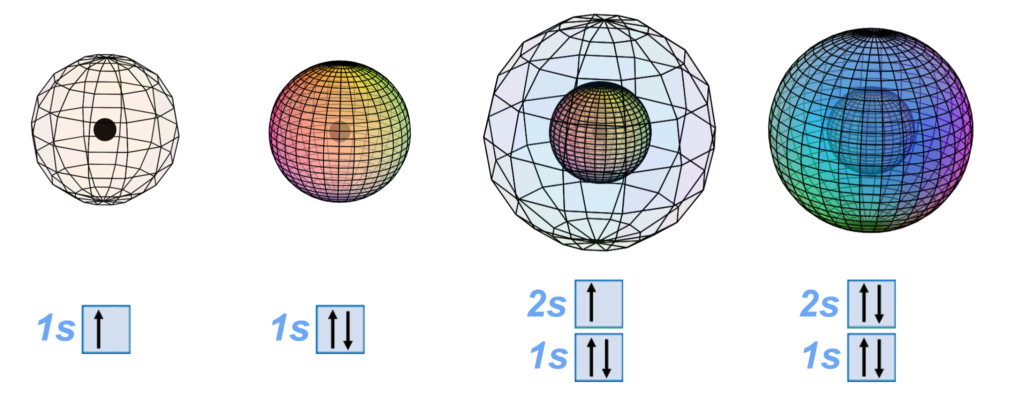Spherical electron clouds of (left to right) hydrogen, helium, lithium and beryllium, along with their orbital diagrams.

Spherical symmetry is lost when the 5th element, boron, adds its 5th electron, and from that point on, atoms must subdivide their electron shells in order to achieve the spherical symmetry required by stable stationary wave structures. When additional electrons compete with the second-shell di-electron for the privilege of approaching the nucleus, the volume of the outer shell must become subdivided in a symmetrical fashion, and electrons will find their positions in the resulting orbital resonance by submitting to the relative strengths of their interactions. (See Hybridization below.) Electron orbital geometry is therefore a function of distance from the nucleus, as well as charge (vector), field (bi-vector), and spin (tri-vector) interactions.

TOTAL Di-ELECTRON INCLUSION (TDI):
How is it possible for electrons to pair up when they have the same charge, since like charges repel? In fact, they pair up so forcefully that their pairing forms the covalent bonds that hold atoms together in molecules.

When two electrons of opposite spin envelop a nucleus, they will snap into an antiparallel relationship because this allows them to superimpose completely upon one another. This causes their magnetic fields to be oppositely aligned at every point, resulting in maximum magnetic field cancellation, which lowers both energy and angular momentum significantly. At such close distances, the attraction of magnetic field cancellation overwhelms electrostatic charge repulsion since the magnetic force is about two orders of magnitude (102) stronger than the electric force at very close range.

This attraction, named Total Di-Electron Inclusion (TDI), gives rise to the di-electron state that is the helium (He) atom’s 1s2 shell. This di-electron is a boson state, which is a distinct state from that of two individual electrons (which are fermions).

When two electrons on adjacent atoms have antiparallel spin, they can be attracted into this superimposed di-electron state, giving rise to a single covalent bond, as in the hydrogen molecule (H2). The lowering of energy from magnetic field cancellation is the attractive force that holds the atoms together. The two proton nuclei remain suspended within the di-electron by the attraction of opposite-charge electric field cancellation, but they remain a distance apart from one another due to the field intensification of like-charge proton repulsion. A molecule can thus be thought of as a discrete crystal-like unit — positive cores suspended in a 3-dimensional “electron gas.”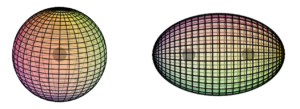Helium’s 1s2 di-electron (left) and the hydrogen molecule’s bonding di-electron (right)

THE PAULI EXCLUSION PRINCIPLE:
If the spherical s-orbitals are full, new electrons must resonate as non-spherical p-orbitals (or higher). This no longer allows the electron density to achieve spherical symmetry around the spherical atomic core. Since the electrons can no longer superimpose as a result of geometric constraints, asymmetry raises resonance energy and decreases coherence. Electrons therefore seek symmetry by venturing into the slightly higher energy state of parallel spin.

Since they are no longer at immediately close range, electrostatic repulsion returns to primacy. This is because the stronger (dipole) magnetic force drops off as the inverse cube (1/r3) of distance whereas the (monopole) electric charge drops off as the inverse square (1/r2) of distance. This means that, since the electric force drops off more slowly than the magnetic force, it is stronger at distance.

Since their magnetic fields no longer cancel perfectly, the increase in energy from constructive magnetic interference causes the electrons to repel each other in search of a lower energy state. In addition, quantum spin density is increased in the region between the electrons.

The sum of these interactions causes the electrons to move as far from each other as their atomic geometry will allow.

However, the energy of these electrons is now higher than it would be if they were in a superimposed, di-electron state (under TDI). This necessitates a lowering of energy in order to compensate, and this is possible through the following quantum spin interactions.

PARALLEL SPIN BONDING (PSB):
According to Sub-Quantum Chemistry, a new theory of electron quantum coherence, once electrons with parallel spin achieve the maximum allowed distance from one another, the forces of field repulsion are in balance. The electrons will then be able to link their spin states into a single, larger, coherent, multi-electron quantum spin state. This lowers energy and pseudo-stabilizes these otherwise unpaired electrons.

Degenerate electrons that are unable to superimpose therefore preferentially assume parallel spin states, creating a more favorable energy condition, with spin bonding, out of one that is otherwise less stable than the preferred (antiparallel) di-electron state. This is what occurs during hybridization. In the sp2 hybridization of boron (B), which we will discuss further below, the 2s2 electrons uncouple from their di-electron state and elevate their energies, so that the single p-electron can lower its energy. The three electrons can then find symmetry and coherence as a set of trigonal planar, degenerate, spin-bonding electrons — a tri-electron state. Spin bonding compensates for the energy increase of electrostatic repulsion by lowering energy through the stabilization of quantum angular momentum linkage. If their spin can be considered radial, the boron valence shell will be a spin=0 region that is nowhere without spin.

The Pauli Exclusion Principle thus embodies the process of field exclusion in concert with Parallel Spin Bonding (PSB) in order to create the next most favorable energy state after the di-electron state.

ELECTRON-Di-ELECTRON REPULSION:
When both unpaired electrons and di-electrons are present in the same shell, di-electrons will repel more strongly than unpaired electrons. This occurs due to the significant lowering of energy from field cancellation that takes place during di-electron formation, but this energy is raised if another field is allowed to interact (and interfere) with it. This is the reason diamagnetic substances repel magnetic fields, and di-electrons will similarly repel the fields of nearby unpaired electrons more strongly than unpaired electrons will repel one another.

As a consequence, electron orbital geometry will be dominated by di-electron geometry, and unpaired electrons will be repelled into secondary (symmetrical) positions as a result.

## Orbitals & Orbital Hybridization

ELECTRON ORBITALS:
When multiple electrons interact in the same atom or molecule, they produce orbital regions that are symmetrical, phase-locked, resonant, coherent, spherically-harmonic, stationary electron waves. Each section or ‘tuft’ within a shell is a region of orbital electron density within this resonance that is typically occupied either by a single electron or a di-electron. The resulting geometry is therefore a consequence of nuclear attraction, mutual electron and di-electron repulsion, and quantum electron interactions. When an electron shell contains multiple orbital sections, the like-charge repulsion between them causes them to separate as much as possible in order to minimize repulsion. This requires spherical symmetry, and Platonic geometry provides us with a foundation on which to consider it. Orbitals will stray from perfect Platonic symmetry, however, when they contain either an odd number of electron domain directions or a combination of unpaired electrons and di-electrons, since di-electrons contain more electron density and repel more strongly than single electrons.Examples of platonic, spherically symmetrical orbitals (L to R): spherical, tetrahedral, octahedral, cubic, cuboctohedral, and the orbitals of krypton (Kr)

The wave structures that are the orbitals are separated by nodes of low (though non-zero) electron density, as seen in the orbital resonances (shown above). Each orbital can therefore be thought of as being confined to its symmetrical volume by fixed nodal boundaries, though they all form a part of a continuous spherical resonance structure — a phase-locked spherical stationary wave — that surrounds the nucleus in the most symmetrical, stable energy state it can achieve. (An analogy in the linear case might be a resonating guitar string, where the symmetrical tufts (or bulges) in the wave appear to remain fixed in location and are separated by nodal points of low amplitude.

Each electron and each di-electron occupies one discrete region within (and at the same time constitutes) this single coherent atomic (or molecular) resonance. This implies that electrons need not “orbit” the nucleus at all in order to remain connected to it. (Spin is an internal electron property. It does not arise from orbiting the nucleus.) On the other hand, a more accurate way to consider this state might be to focus on the energy flow within the coherent harmonic system, rather than considering the elements of it as discrete particles. It is, in fact, their lack of perfect discreteness that allows them harmonic coherence. Whether this means that the photons making up these subatomic particles coalesce into a single greater photonic state or that they remain discrete particles in a perfectly symmetrical geometric dance makes no material difference to the resulting configuration.

Adding to or removing electrons (or photons) from this atomic resonance state causes it to snap into the next (either higher- or lower-energy) stable resonance state. The difference in energy between these resonance states represents the amount of energy arriving or leaving the atom during the transition.

ORBITAL HYBRIDIZATION:
Electrons strive to maximize symmetry and minimize repulsion. The way that orbitals achieve this is known as hybridization. It is when orbitals — that we normally designate as having different energies and geometries, like s– and p-orbitals — combine and rearrange themselves into a symmetrical arrangement of identical energy states. Overall, this symmetrical state has a lower energy than the sum of the individual energy states before they hybridized.

Hybridizations like sp, sp2, sp3, sp3d, and sp3d2 are familiar concepts. The first instance of it occurs in the boron atom with sp2-hybridization. With five electrons, boron is the first atom to contain electrons that are in a p-orbital. This is not a sphere-shaped harmonic, and so a single electron in a p-orbital cannot find a symmetrical arrangement around a sphere by itself. Boron therefore cannot simply add its p-electron on top of the same (2s2) configuration that beryllium has, as shown below, because it would not be stable:Each p-orbital lobe can hold 1 electron. An electron pair occupies two opposite lobes.

The asymmetry therefore causes the two electrons in the 2s orbital to uncouple from their di-electron state and form a tri-electron state with the single p-electron. Three equal-energy (degenerate) electrons can now achieve maximum stability by assuming a trigonal planar (sp2-hybridized) arrangement around the core electron shell, because this minimizes their mutual repulsion by having them as far from one another as they can get. (The wireframe indicates the boundary of the n=2 shell.)

The outer spheres in the image above (on the right) simply indicate the directions of maximum electron density. The orbitals themselves will be more like three equal longitudinal sections. Each orbital occupies one third of the volume of the shell, though not uniformly, as electron density has a maximum at the center of the orbital and decreases towards the orbital boundary. But electron density will nonetheless fill the entire shell in order to shield the positive charge of the nucleus. In the case of boron, each of the three hybrid orbitals contains one electron.Boron’s three 2sp2-orbitals surrounding a 1s2 core di-electron shell (left), with top view (right).

In the case of carbon (C), the sp3-hybridization that occurs allows the atom to achieve tetrahedral symmetry involving the s-orbital and three p-orbitals of the 2nd shell. Carbon’s electron configuration is 1s22s22s2. Like boron, carbon cannot simply add its two p-electron into the same (2s2) configuration that beryllium (Be) has, as shown below, because it would not be stable:

The asymmetry therefore causes the two paired electrons in the 2s orbital to uncouple from their di-electron state in order to form a tetra-electron state with the two p-electrons. The four equal-energy (degenerate) electrons can now achieve maximum stability by assuming a 4-directional (tetrahedral, sp3-hybridised) arrangement around the core electron shell. This minimizes repulsion by having them as far from one another as they can get.The orbital diagram for carbon. The wireframe (on the right) indicates the boundary of the 2nd shell.

The small spheres within the 2nd shell in the image above (right) simply indicate the directions of maximum electron density. The orbitals themselves will be more like four equal tetrahedral sections that can only occupy volume within their shell (see below). Each orbital occupies one fourth of the volume of the shell, though not uniformly, as electron density has a maximum at the center of the orbital and decreases towards the orbital boundary due to electron repulsion. But electron density will nonetheless fill the entire shell in order to shield the positive charge of the nucleus in every direction. In the carbon atom, each of the four hybrid orbitals contains one electron.

Four-directional (tetrahedral) symmetry is significant because, after the sphere, it is the first multi-directional symmetry that can attain perfect spherical symmetry. While the three-directional (sp2) symmetry of boron is symmetrical in the equatorial dimension, it is not spherically symmetrical since its axial electron density will be lower than its equatorial electron density.

In the case of neon (Ne), at the end of the second row of the periodic table, there is a full valence shell containing 8 electrons. The traditional view of the full 2nd shell involves a spherical 2s2 orbital di-electron, with 6 p-electrons resonating within it in mutually orthogonal directions (below, left). It is proposed, however, that the most symmetrical and lowest energy state for neon is achieved with 4 di-electrons in spherical tetrahedral symmetry (below, right). The 8 electrons sharing the small second valence shell would achieve lowest energy if they paired up, since field cancellation is maximized in di-electron formation. This also allows the distance between the regions of maximum electron density in the orbital lobes to be the greatest, which minimize repulsion more effectively.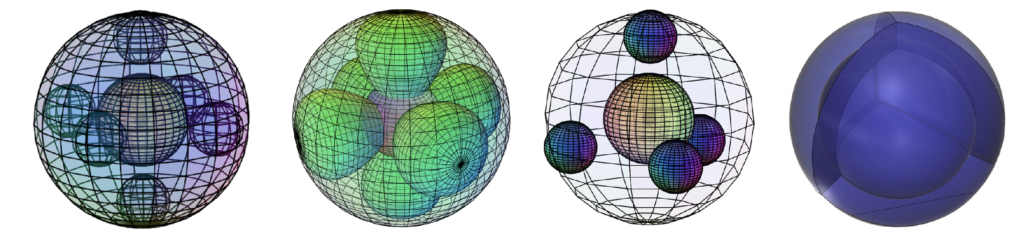The unhybridized version of neon’s orbitals (left) with its traditional lobe view (green), compared to the tetrahedral views of neon’s di-electron orbital symmetry (right)

If that is the case, the electron configuration of argon (Ar) should produce two nested spherical tetrahedra, aligned antiparallel, as shown below.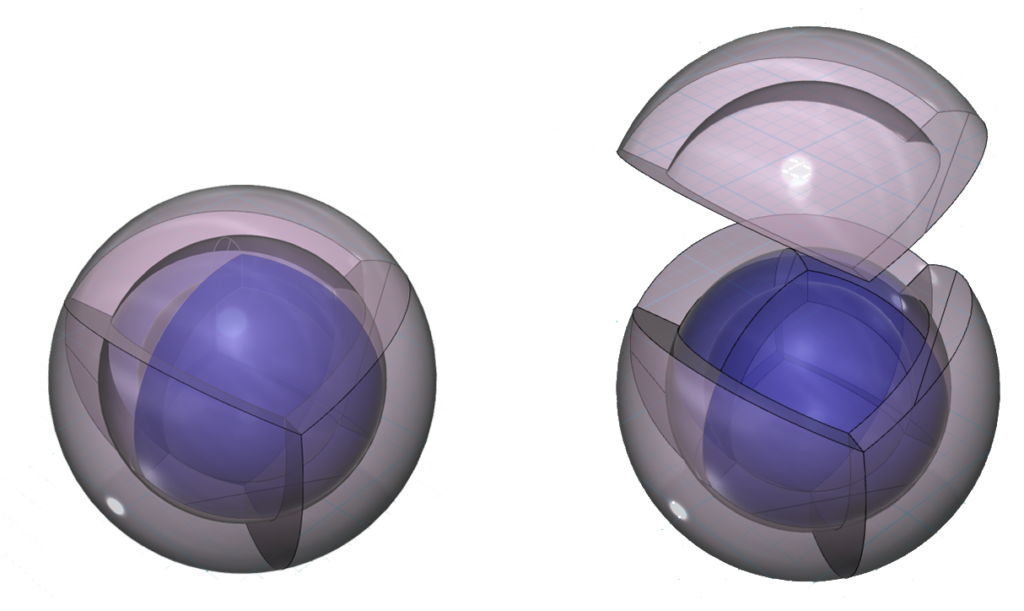Argon’s nested tetrahedral orbital geometry (left), and with one outer orbital raised (right)

The reason that this nested antiparallel tetrahedral geometry is so stable is that the region of lowest electron density on one shell lines up with the region of highest electron density on the adjacent shell, thereby minimizing repulsion. In the diagram on the left above, the vertex where the nodes between orbitals intersect is a point of lowest electron density. It lies directly opposite the center of the face of the orbital above or beneath it, which is the point of highest electron density in that orbital. This overlap is therefore the lowest energy state.

Like oxygen, the sulfur (S) atom contains 6 valence electrons arranged into two di-electrons and two unpaired electrons. An example of sp3d2-hybridisation occurs when a sulfur atom is approached by a highly electronegative atom like oxygen or fluorine. Their high electronegativity can induce one or both of sulfur’s valence di-electrons to uncouple, yielding up to 6 unpaired electrons available for bonding. This is possible for sulfur but not for oxygen because the 3rd shell contains a larger volume than the 2nd shell, which is the reason it can accommodate a d-orbital. While sulfur does not usually have electrons in its 3d-orbital, the 3rd shell has enough volume to accommodate the additional electron density. This allows sulfur’s electrons to hybridize into more electron domain directions than a 2nd row element. Sulfur can therefore make up to 6 covalent bonds, as we see in the octahedral case of sulfur (shown below), as found in the sulfur hexafluoride (SF6) molecule.